### IMO Shortlist 2009 problem C2

Kvaliteta:
Avg: 0,0
Težina:
Avg: 6,0
For any integer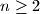$n\geq 2$, let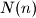$N(n)$ be the maxima number of triples$(a_i, b_i, c_i)$,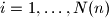$i=1, \ldots, N(n)$, consisting of nonnegative integers$a_i$,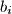$b_i$ and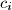$c_i$ such that the following two conditions are satisfied: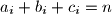$a_i+b_i+c_i=n$ for all$i=1, \ldots, N(n)$, If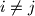$i\neq j$ then$a_i\neq a_j$,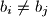$b_i\neq b_j$ and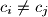$c_i\neq c_j$Determine$N(n)$ for all$n\geq 2$.

Proposed by Dan Schwarz, Romania
Izvor: Međunarodna matematička olimpijada, shortlist 2009